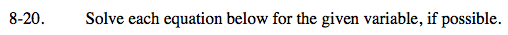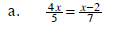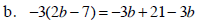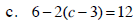### Home > CAAC > Chapter 8 > Lesson 8.1.2 > Problem8-20

8-20.
1. Solve each equation below for the given variable, if possible. Homework Help ✎

1.2. −3(2b − 7) = −3b + 21 − 3b

3. 6 − 2(c − 3) = 12Multiply both sides by 35 to cancel out the denominators.